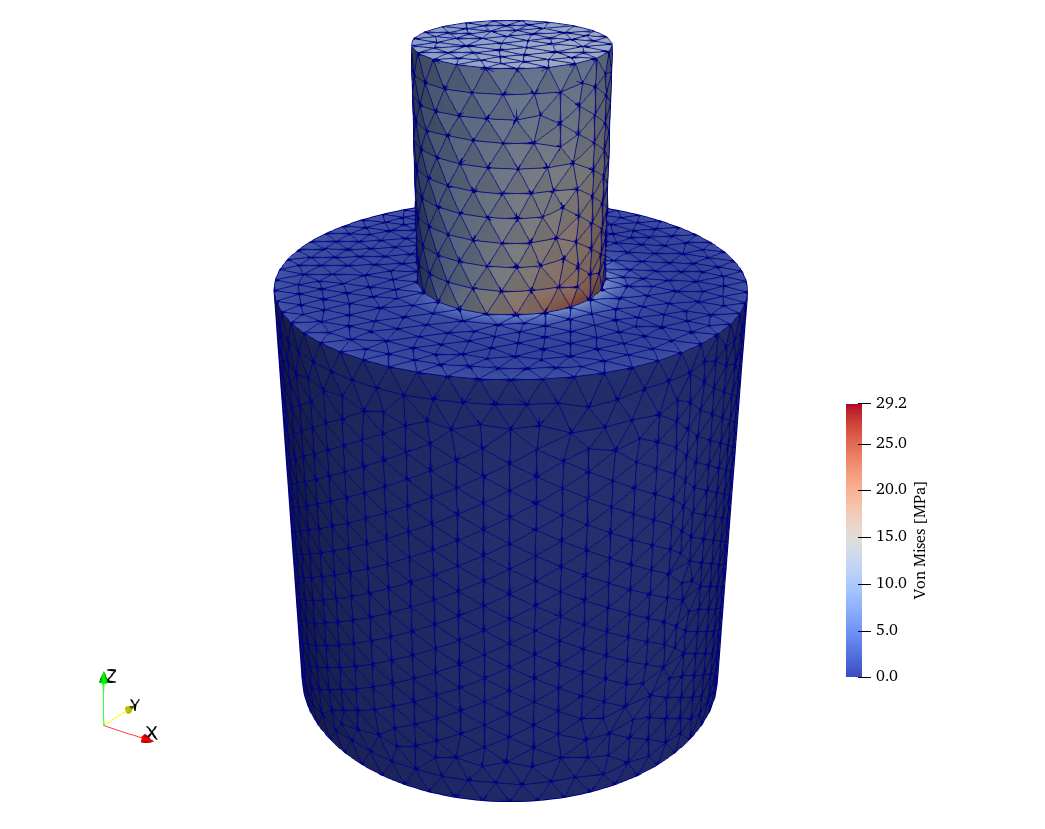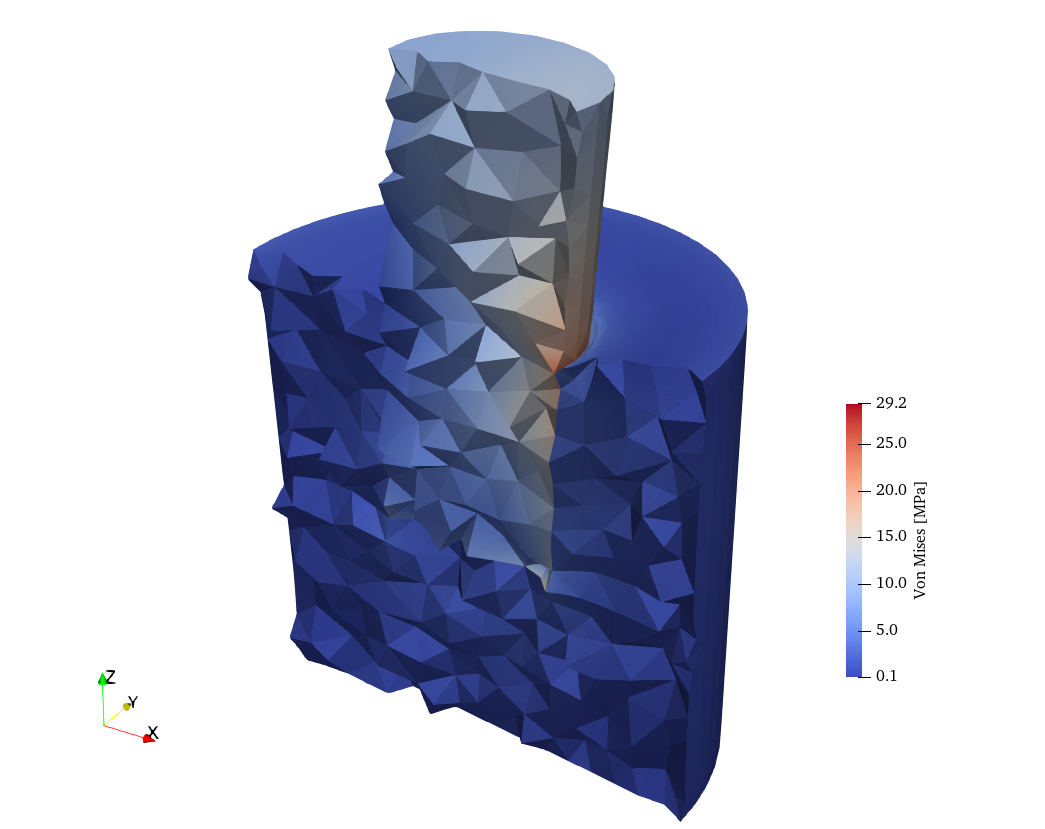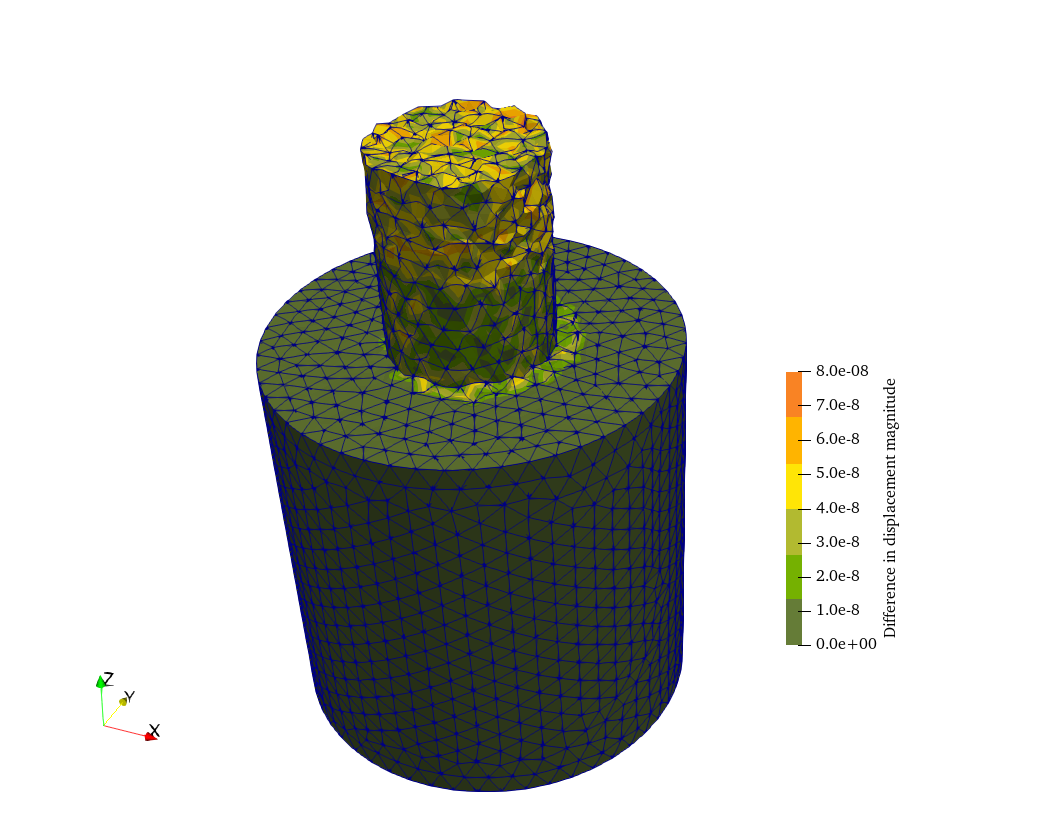# Cylinder embedded in another cylinder—rough

 Title Cylinder embedded in another cylinder—rough Tags elasticity compression bending Runnng time a few seconds See also 050-tet10105-cyl-cyl-smooth070-two-cubes Available in HTML PDF ePub

# 1 Problem description

The continuous problem to be solved is exactly the same as the one solved in Cylinder embedded in another cylinder—smooth. The difference is that this time we

1. use straight (i.e. not curved) tetrahedra so we can compare results with Sparselizard, and
2. ask Fino not to smooth out stresses and to keep the contribution of each element to strains and stresses (see Two cubes of different materials).

# 2 Geometry and mesh

The geometry was created and meshed directly in Gmsh. The following cyl-cyl-rough.geo is similar to the geometry file from Cylinder embedded in another cylinder—smooth except that it

1. creates the geometry using the OpenCASCADE kernel embedded into Gmsh, and
2. asks Gmsh to create straight tetrahedra where the mid-edge nodes are actually at the center of the tetrahedra edges instead of fitting the curved geometry surfaces.
// create the geometry with the OpenCASCADE kernel
Cylinder(1) = {0, 0, 0, 0, 0, 50, 25};
Cylinder(2) = {0, 0, 25, 0, 0, 50, 10};
BooleanDifference{ Volume{1};Delete; }{ Volume{2}; }
Coherence;  // this step creates a single surface for the interface

// define physical groups
Physical Volume("base",1) = {1};
Physical Volume("stem",2) = {2};
Physical Surface("fixed",3) = {9, 7};

// mesh options
Mesh.CharacteristicLengthMax = 3.0;

Mesh.SecondOrderLinear = 1;  // straight
Mesh.ElementOrder = 2;       // second-order elements

Mesh.Algorithm = 6;
Mesh.Algorithm3D = 1;

Mesh.Optimize = 1;
Mesh.OptimizeNetgen = 1;
Mesh.HighOrderOptimize = 1;

Mesh.MshFileVersion = 2.2;  // sparselizard needs the older v2.2

This way, the same .msh file can be used to solve the problem with Fino and with Sparselizard.

# 3 Input file

The Fino input file cyl-cyl-rough.fin is almost identical to the previous case Cylinder embedded in another cylinder—smooth. The only difference is that we now ask Fino to never smooth strains nor stresses with FINO_SOLVER SMOOTH never.

MESH FILE_PATH cyl-cyl-rough.msh DIMENSIONS 3    # load mesh

# assign per-material properties
MATERIAL stem E 120e3  nu 0.26
MATERIAL base E 2.5e3  nu 0.35

# set boundary conditions
PHYSICAL_ENTITY fixed BC fixed

# ask Fino not to smooth stresses
FINO_SOLVER SMOOTH never
FINO_STEP

# write output as in previous case
MESH_POST FILE_PATH cyl-cyl-rough-fino.vtk VECTOR u v w sigma
# write another output for comparison with sparselizard
MESH_POST FILE_PATH fino-sigma.vtk sigma

# report maximum diplacement in scientific notation
PRINT "Maximum displacement magnitude:" %e displ_max "mm"

# 4 Execution

$gmsh -v 0 -3 cyl-cyl-rough.geo$ fino cyl-cyl-rough.fin
Maximum displacement magnitude: 2.526434e-02    mm
$ # 5 Results As the input file is almost exactly equal to the previous problem, the output is also similar (fig. 1). The difference is that stresses are not smoothed out so a “rough” plot is obtained. As expected, the maximum stress is higher. Also, all the triangles that compose the tetrahedra are co-planar.ab Figure 1: Von Mises stresses obtained by Fino. a — Full view, b — Clipped & warped \times 250 view # 6 Check We now solve the same problem with Sparselizard. The following main.cpp file is used: #include "sparselizardbase.h" using namespace mathop; void sparselizard(void) { int base = 1; int stem = 2; int fixed = 3; int load = 4; int bulk; formulation elasticity; mesh mymesh("cyl-cyl-rough.msh"); field u("h1xyz"); bulk = regionunion({1,2}); u.setorder(bulk, 2); u.setconstraint(fixed); parameter E, nu, lambda, mu; E|base = 2.5e3; E|stem = 120e3; nu|base = 0.35; nu|stem = 0.26; lambda|bulk = E*nu/((1+nu)*(1-2*nu)); mu|bulk = 0.5*E/(1+nu); elasticity += integral(bulk, predefinedelasticity(dof(u), tf(u), E, nu)); elasticity += integral(load, array1x3(+1,0,-10)*tf(u)); solve(elasticity); expression H(6,6,{lambda+2*mu, lambda, lambda, 0, 0, 0, lambda, lambda+2*mu, lambda, 0, 0, 0, lambda, lambda, lambda+2*mu, 0, 0, 0, 0, 0, 0, mu, 0, 0, 0, 0, 0, 0, mu, 0, 0, 0, 0, 0, 0, mu}); expression stress = H*strain(u); vonmises(stress).write(bulk ,"lizard-sigma.vtk", 2); u.write(bulk, "lizard-displ.vtk", 2); return; } int main(void) { SlepcInitialize(0,{},0,0); sparselizard(); SlepcFinalize(); return 0; }  The displacement vector field can be recovered back by Fino/wasora and compared to the Fino solution, as in Cylinder embedded in another cylinder—smooth. Indeed, fig. 2 shows that these differences are in the range of 10^{-8}~\text{mm}, which is related to the precision of the ASCII representation both codes use to write results in VTK format. MESH NAME fino FILE_PATH cyl-cyl-rough-fino.vtk DIMENSIONS 3 { READ_SCALAR u_v_w1 as u READ_SCALAR u_v_w2 as v READ_SCALAR u_v_w3 as w } MESH NAME lizard FILE_PATH lizard-displ.vtk DIMENSIONS 3 { READ_SCALAR lizard1 AS u_l READ_SCALAR lizard2 AS v_l READ_SCALAR lizard3 AS w_l } diff_u(x,y,z) := u_l(x,y,z) - u(x,y,z) diff_v(x,y,z) := v_l(x,y,z) - v(x,y,z) diff_w(x,y,z) := w_l(x,y,z) - w(x,y,z) MESH_POST MESH fino FILE_PATH diff-rough-fino.vtk VECTOR diff_u diff_v diff_w MESH_POST MESH lizard FILE_PATH diff-rough-lizard.vtk VECTOR diff_u diff_v diff_wFigure 2: Absolute difference in the displacement fields computed by Fino and Sparselizard However, the stresses cannot be directly computed as the algebraic difference of two spatial functions \sigma(x,y,z) as these functions are not well-defined. In effect, for each node (that has an unique spatial coordinate \vec{x} = [ x,y,z ] which is the independent variable) there are as many dependent variables as elements sharing said node, i.e. up to ten different values. Hence, the operation “difference” cannot be performed at the node—even though it is inside each element. We might take a shot at directly comparing the VTK file but it turns out that Fino and Sparselizard handle the mesh data in different ways, so the mesh topology stored in the VTK file is different. One of Sparselizard’s killer features is automatic p-refinement so elements are stored and written in the output file in a particular way. Nevertheless, we can check that the results obtained with both programs are essentially the same as follows. First, we extract the numerical data of the von Mises stress from the VTK file with the following AWK script. { if ($1 == "POINT_DATA") {
do {
getline
} while ($1 != "LOOKUP_TABLE"); getline flag = 1 } if (flag &&$0 != "") {
print $0 } }  After applying this filter to both VTK files, we get different values: $ awk -f extract.awk fino-sigma.vtk  | head
3.53101
0.714683
1.2371
1.49287
1.92684
0.924515
2.25453
2.50164
1.2529
0.930675
$awk -f extract.awk lizard-sigma.vtk | head 0.085090400929917029 0.088449441668799159 0.23575407201125495 0.12823643224463696 0.086490009161928866 0.161958081221071 0.16023216465718956 0.10309986345574841 0.17704267927878406 0.10479619158456339$ 

But, if—as suggested by having almost the same displacement field (fig. 2)—the stresses are also almost equal, sorting these two outputs numerically should lead to similar outputs now:

$awk -f extract.awk fino-sigma.vtk | sort -g | head 0.064166 0.0652736 0.0661323 0.0662491 0.06626 0.0662741 0.0665915 0.0671003 0.0673532 0.0673678$ awk -f extract.awk lizard-sigma.vtk  | sort -g | head
0.064165938666383981
0.065273696128195044
0.066132586533121374
0.066249723539956784
0.066259947259440066
0.066273896515029324
0.066591525065027551
0.067100091418163504
0.067353077383710935
0.067367862498671632

To make sure this similarity holds for the whole data set we paste these two outputs together, take the numerical difference and sort the differences again to see what the maximum is:

$for i in fino lizard; do awk -f extract.awk${i}-sigma.vtk | sort -g > ${i}-sorted.dat; done$ paste lizard-sorted.dat fino-sorted.dat | awk '{print $1-$2}' | sort -g  | head -n1
-0.000167408
$paste lizard-sorted.dat fino-sorted.dat | awk '{print$1-$2}' | sort -gr | head -n1 0.000193749$

This means that there are no more than 0.2 \times 10^{-4}~\text{MPa} of difference between Fino and Sparselizard. That is 0.2 pascals, which might be also related to the different precision both programs write the decimal ASCII representation of the actual floating-point values stored in the computer’s memory. Hence, even using different approaches, it is clear that both Fino and Sparselizard end up solving the same set of equations for the linear elastic problem—including multi-solid geometries—provided that

1. the elements used by Fino are “straight” and not “curved” (see Stresses in a 10-node tetrahedron with prescribed displacements), and
2. Fino is asked to never average strains and stresses (a.k.a. “rough mode”—see Two cubes of different materials).